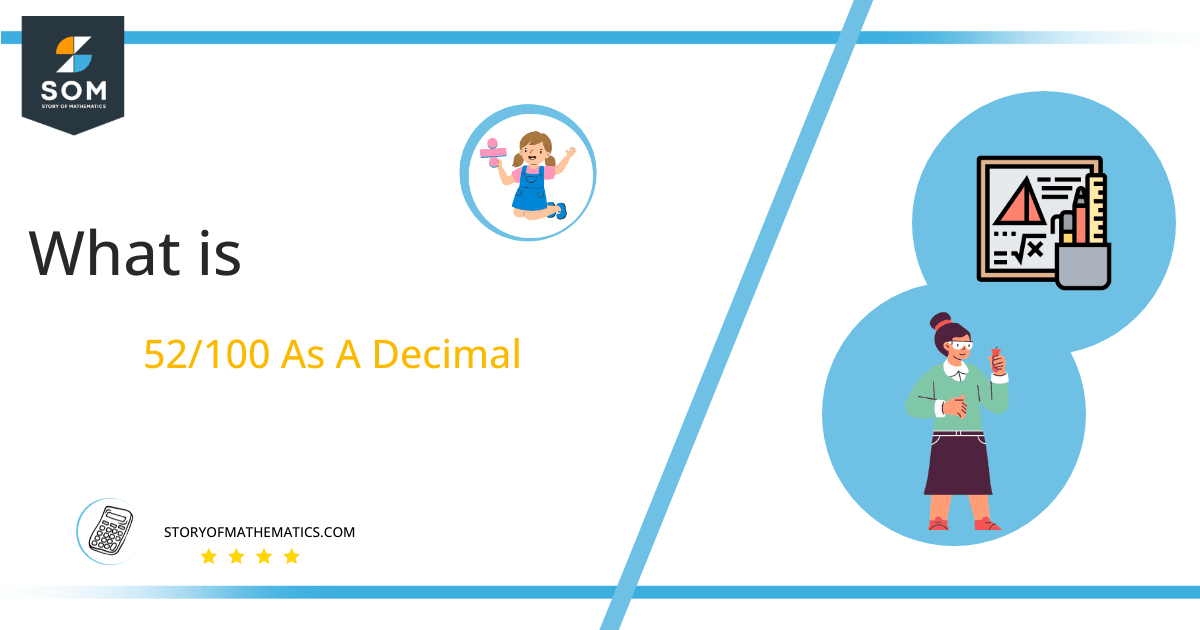# What Is 52/100 as a Decimal + Solution With Free Steps

The fraction 52/100 as a decimal is equal to 0.52.

Fractions are converted into Decimal values to make them easier to understand, and decimal values are more useful in mathematical problems. Fractions can be represented in p/q form, where p and q stand for Numerator and Denominator, respectively.

Here, we are more interested in the division types that result in a Decimal value, as this can be expressed as a Fraction. We see fractions as a way of showing two numbers having the operation of Division between them that result in a value that lies between two Integers.Now, we introduce the method used to solve said fraction to decimal conversion, called Long Division, which we will discuss in detail moving forward. So, let’s go through the Solution of fraction 52/100.

## Solution

First, we convert the fraction components, i.e., the numerator and the denominator, and transform them into the division constituents, i.e., the Dividend and the Divisor, respectively.

This can be seen done as follows:

Dividend = 52

Divisor = 100

Now, we introduce the most important quantity in our division process: the Quotient. The value represents the Solution to our division and can be expressed as having the following relationship with the Division constituents:

Quotient = Dividend $\div$ Divisor = 52 $\div$ 100

This is when we go through the Long Division solution to our problem.Figure 1

## 52/100 Long Division Method

We start solving a problem using the Long Division Method by first taking apart the division’s components and comparing them. As we have 52 and 100, we can see how 52 is Smaller than 100, and to solve this division, we require that 52 be Bigger than 100.

This is done by multiplying the dividend by 10 and checking whether it is bigger than the divisor or not. If so, we calculate the Multiple of the divisor closest to the dividend and subtract it from the Dividend. This produces the Remainder, which we then use as the dividend later.

Now, we begin solving for our dividend 52, which after getting multiplied by 10 becomes 520.

We take this x1 and divide it by y; this can be seen done as follows:

520 $\div$ 100 $\approx$ 5

Where:

100 x 5 = 500

This will lead to the generation of a Remainder equal to 520 – 500 = 20. Now this means we have to repeat the process by Converting the 20 into 200 and solving for that:

200 $\div$ 100 = 2

Where:

100 x 2 = 200

Finally, we have a Quotient generated after combining the two pieces of it as 0.52 = z, with a Remainder equal to 0.Images/mathematical drawings are created with GeoGebra.